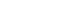# Mathematical Thinking on the GMAT

What does the GMAT Quantitative section test?  Math, of course.  As tautological as that may seem, there is a very subtle point here.  What exactly is math?

## Mathematics

Strange as it may seem, there is no generally accepted formal definition of the word mathematics.  Most of the dictionary definitions, for example, cover the more familiar branches of mathematics, but not, say, typical Ph.D. dissertation topics in math.  One aspect of math has to do with logical relationships and logical deductions — we could call this the more left brain part of math.  Another aspect of math has to do with identifying formal abstract patterns.   The words “logic” and “patterns” give two very important clues.  For the first, you really understand a mathematic topic when you understand all the logical connections —- for example, knowing not just the rule for adding fractions, but understanding why fractions have to be added that way.   The second is relevant because several out-of-the-box GMAT questions will throw some new situation at you, and you will have to identify what is the best way to parse the situation into recognizable components.   Perhaps even more important is understanding what math is not.

## Mathematics and the GMAT Math Review

I believe if one were to ask many a GMAT test taker, “What math does one need to know for the GMAT?”, most people would indicate the content of the OG, the section entitled “Math Review.”  This notion is simultaneously very helpful but slightly misleading.   Yes, those are the math facts you need to know — and if you’re rusty on them, as many folks are at the beginning of studying, it’s good to get very clear on them — but the math facts are not the same as mathematics.  What’s the difference?

Well, think of the difference between, say, the content of a French dictionary vs. the language of French in living dialogue.  As helpful as a dictionary may be, no one goes from no knowledge of the language to fluency simply by relying on the dictionary.   In addition to knowing what each word means in isolation, one also has to know how it is used, in combination, in the context of real live communication.  In this analogy, the “Math Review” communicates the meaning of each individual math factoid, and knowing these is essential, but the mathematics one really has to know is how to use these in problem-solving.  If this is what  you need to learn, you only find it by solving problem after problem, and carefully reading the solutions of each problem.

When you read the solutions to a problem that baffled you, pay attention to (a) the logic of the argument connecting one step to the next — not just the math factoids, but the strategies employed, and (b) the patterns the solution points out and employs.   Any logic that is new to you or any patterns you didn’t see on your own — make a note of these, and return to them until you are confident you would be able to use these in the solution of a new problem on your own.

## Formulas

Much in the same vein, a student can easily lean on formulas too much, and mistake this reliance on formulas for mathematical thinking.   Don’t memorize the formula for the sum of the first n integers.  Don’t memorize the formula for the area of an equilateral triangle.  Don’t memorize the formula for distance in the coordinate plane.  Yes, those are all things you may need to calculate in a GMAT question, but instead of memorizing a formula, I highly recommend understanding, step by step, the logical argument that underlies the formula.   Knowing the formula, by itself, is not mathematical thinking, but understanding the logic, the argument, that leads to the formula — that most certainly is mathematical thinking.

There are very few formulas you should simply memorize.  One that leaps to mind is Archimedes‘ formula for the area of a circle.Archimedes was one of the greatest mathematical geniuses of all time, and  he concocted a truly brilliant argument for why this formula had to be true.  That argument, fascinating as it may be, is a bit more math than I would recommend for the average GMAT preparer.  On this one, I would say: take a pass — just memorize this formula by rote, if you don’t already have it memorized from school.  Having said that, I can’t think of any other GMAT formula about which I unreservedly would say the same thing.  For every other formula, do not settle for rote memorization.  Strive to understand the underlying logic.

## Summary

St. Paul  wrote “the letter killeth but the spirit giveth life” (2 Cor. 3:6).  While this is arguably an over-dramatic analogy in context here, the sense of it holds.  Mathematical thinking is a vital fluency with math, and you won’t find it printed on the page of the GMAT “Math Review.”  What’s on those pages is necessary but not sufficient.  Here are a few problems, for practicing mathematical thinking.

## Author

•Mike served as a GMAT Expert at Magoosh, helping create hundreds of lesson videos and practice questions to help guide GMAT students to success. He was also featured as "member of the month" for over two years at GMAT Club. Mike holds an A.B. in Physics (graduating magna cum laude) and an M.T.S. in Religions of the World, both from Harvard. Beyond standardized testing, Mike has over 20 years of both private and public high school teaching experience specializing in math and physics. In his free time, Mike likes smashing foosballs into orbit, and despite having no obvious cranial deficiency, he insists on rooting for the NY Mets. Learn more about the GMAT through Mike's Youtube video explanations and resources like What is a Good GMAT Score? and the GMAT Diagnostic Test.